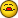Average Non-consecutive Cells

D

dave.melvin

Been trying to figure this one out...

I track several parameters month/quarterly/annually in this fashion:

| Jan | Feb | Mar | Q1 | Apr | May | Jun | Q2 | Jul | Aug | Sep | Q3 |
Oct | Nov | Dec | Q4 | Annual |

The Quarterly columns are the average of the preceding three months.
This part works fine with {=AVERAGE(IF(A154:C154<>0,A154:C154,0))} in
Q1 for example. I use the array to eliminate a skew by future numbers,
i.e., it only averages non-zero cells.

Here's my quandary - to get the same functionality in the "Annual"
column, currently I'm placing the values from Q1,Q2, Q3 and Q4 in
contiguous cells elsewhere in the spreadsheet and using the same type
of array formula in the "Annual" cell on that block.

Is there a way to eliminate this step? The current setup just doesn't
seem elegant to me. I can't seem to figure out a formula that I can
place in "Annual" that will average the non-zero values from Q1,Q2, Q3
and Q4 since they aren't consecutive cells.

Dave

B

Bob Phillips

=AVERAGE(D154,H154,L154,P154)

or if that doesn't work, then

=AVERAGE(IF((NOT(ISERROR(A154154)))*(MOD(COLUMN(A154154),4)=0),A154154))

--
HTH

Bob

(there's no email, no snail mail, but somewhere should be gmail in my addy)

G

Gary''s Student

AVERAGE() ignores blanks, but includes zeros. Change the quarter formulas to
something like:

=IF(old_quarter_formula=0,"",old_quarter_formula)

The yearly average will not be skewed by incomplete data. By the way, you
can do something similar for the quarter formulas themselves.

G

GerryGerry

You could use the following:
=SUM(D154,H154,L154,P154)/SUM(COUNTIF(INDIRECT({"D154","H154","L154","P154"}),">0"))

D

dave.melvin

You could use the following:
=SUM(D154,H154,L154,P154)/SUM(COUNTIF(INDIRECT({"D154","H154","L154","P154"}),">0"))

GerryGerry,

Many thanks - this works like a charm!

Dave